# Tank Volume Calculator

Use this tank volume calculator to easily calculate the volume of a water tank, oil tank, fuel tank, and so on. The tank size calculator supports a dozen different tank shapes: cylinder, rectangle, oval, capsule, elliptical, etc. and outputs tank volume (cu ft, cu m) and liquid volume (US/UK gallons, litres, BBL).

Share calculator:

Embed this tool:
get code

## Using the Tank Volume Calculator

This tank calculator is a versatile tool allowing to calculate tank volume (a.k.a. tank capacity), liquid volume, and the volume of the liquid currently in the tank. The calculator is useful in planning tank capacity in construction projects, water purifier plants, oil storage systems, and others.

To use the calculator, simply select the shape of the tank, then fill in the respective dimensions, then click calculate to see the results. You can also enter the height of the liquid currently present in the tank to get an estimate of its volume, liquid volume, and the percentage of the tank capacity currently in use. When filling the dimensions, make sure to use the inner dimensions of the tank. If these are not available, you should make sure to account for the thickness of the tank walls in your volume calculations.

It should be noted that all calculations should be treated as estimates for real-life purposes since they assume certain perfection in the shape of the tank - e.g. a perfect half-sphere for domes and perfect half-cylinders for ovals. Also, real tanks might have tubing or detectors on the inside which might take space not accounted for in the output of this tool.

Supported tank measurements are in mm, cm, dm, meters, inches, feet, and yards. The tank volume calculator will output the volume in cubic meters and cubic feet and used capacity in the same metrics. It will also output liquid maximum volume in litres (liters), US gallons, UK gallons, and BBL (US Oil barrels) and similarly for the liquid volume of the liquid in the tank. It will also output the percentage of the tank capacity in use based on the liquid level (liquid height) entered.

### Supported tank shapes

Below is a list of tank shapes supported by the calculator. It contains notes regarding important assumptions and illustrations of the necessary dimensions for volume calculations for each shape:

Tank shapes supported in the calculator
Tank shapeIllustration with required dimensions
Rectangular tank (rectangular prism)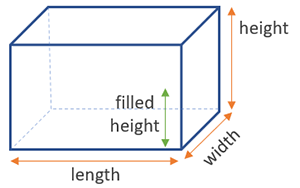Horizontal cylinder tank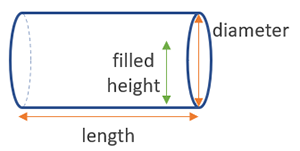Horizontal cylinder tank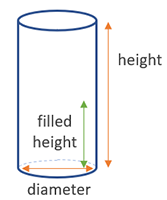Horizontal capsule tank
(note: calculation presumes each end is half a sphere)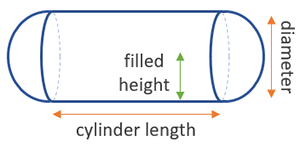Vertical capsule tank
(note: calculation presumes each end is half a sphere)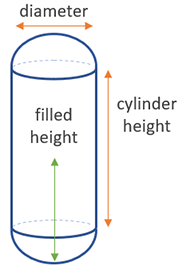Vertical elliptical tank (oval tank)
(note: calculation presumes each end is half a cylinder)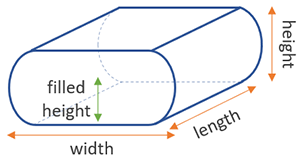Vertical elliptical tank (oval tank)
(note: calculation presumes each end is half a cylinder)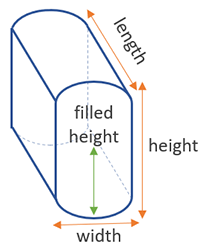Cone bottom tank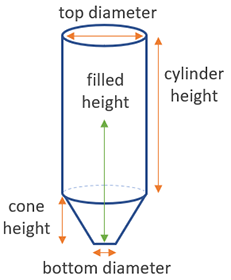Cone top tank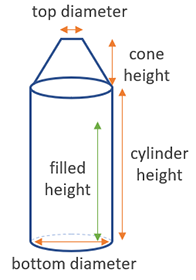Dome top tank
(note: calculation presumes the dome is half a sphere)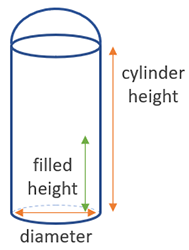Dome top cone bottom tank
(note: calculation presumes the dome is half a sphere)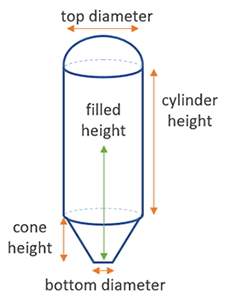Dome top oblique cone bottom tank
(note: calculation presumes the dome is half a sphere)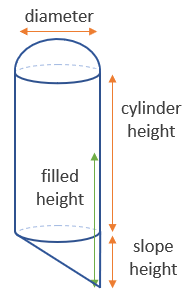Oblique cone bottom tank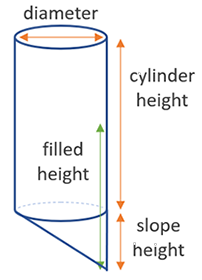Note that the tool does not differentiate tanks based on their purpose or the liquid they contain. It can be used as a water tank calculator, oil tank calculator, fuel tank calculator, and so on.

## How to calculate the volume of a tank?

For simple tanks such as rectangular prisms or cylinders the calculation is straightforward and exactly the same as the ones used in our cylinder volume and box volume calculators. For complex shapes such as dome tops, cone tops or bottoms, oblique cone bottom, ovals, and capsules, the tank volume is calculated as a sum of the component simpler shapes.

For example, the volume of a capsule tank is calculated as the sum of a sphere and a cylinder, whereas a cone bottom tank's capacity is estimated by summing the volume of the cone and the volume of the cylinder. Similarly, a dome top tank's volume is calculated as the sum as the volume of a half-sphere and a cylinder. The usual volume formulas for these figures are used. Obviously, if using our tank capacity calculator all this work is performed automatically for you.

## Calculating the volume of a liquid in a tank

The volume (in m3 or ft3) and liquid volume (in gallons or litres) of a liquid poured in a tank can be calculated easily assuming the tank is sitting with its bottom perpendicular to the ground surface (it is not tilting) and there is a way to measure the liquid level. If the tank has no visible level meter or gauge and it is safe to open it, its level could be measured by descending a clean and dry rod to the bottom of the tank, then retracting it and measuring the level the liquid reached. Note that this is easier to do with oils compared to water.

The filled volume calculation itself usually consists of adding up parts of the tank volume depending on how high the liquid level is. For example, for a cone bottom tank, if the level is below the cone edge one doesn't need to consider the cylinder part of the tank at all. For simple tanks such a cylinder tank, one simply needs to replace the tank height with the observed liquid height in the usual volume of a cylinder equation. The resulting filled volume can easily be converted to a percentage of the total tank capacity as calculated by our calculator or as specified by the manufacturer specifications.

#### Cite this calculator & page

If you'd like to cite this online calculator resource and information as provided on the page, you can use the following citation:
Georgiev G.Z., "Tank Volume Calculator", [online] Available at: https://www.gigacalculator.com/calculators/tank-volume-calculator.php URL [Accessed Date: 27 Mar, 2023].